# Solutions of Refraction of Light (Page No- 240) - Physics By Lakhmir Singh, Class 10 Class 10 Notes | EduRev

## Class 10 : Solutions of Refraction of Light (Page No- 240) - Physics By Lakhmir Singh, Class 10 Class 10 Notes | EduRev

The document Solutions of Refraction of Light (Page No- 240) - Physics By Lakhmir Singh, Class 10 Class 10 Notes | EduRev is a part of the Class 10 Course Class 10 Physics Solutions By Lakhmir Singh & Manjit Kaur.
All you need of Class 10 at this link: Class 10

Lakhmir Singh Physics Class 10 Solutions Page No:240

Question 9: For what position of an object a real, diminished image is formed by a convex lens ?

Solution : Beyond 2F.

Question 10: If an object is at a considerable distance (or infinity) in front of a convex lens, where is the image formed ?

Solution : At focus F.

Question 11: Draw the given diagram in your answer book and complete it for the path of a ray of light after passing through the lens.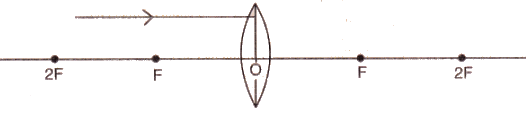Solution :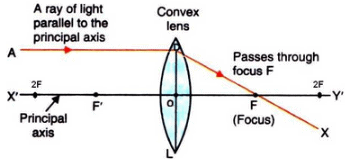Question 12: What type of lens would you use as a magnifying glass ? How close must the object be to the lens ?

Solution : Convex lens

The object must be withing the focus of the lens.

Question 13: Name two factors on which the focal length of a lens depends.

Solution : Focal length of a lens depends on the refractive index of the glass from which it is made, and on the curvature of its two surfaces.

Question 14: State any two uses of convex lenses.

Solution : Two uses of convex lenses:-
1. As a magnifying glass.
2. For making a simple camera.

Question 15: Fill in the following blanks with suitable words :
(a) Parallel rays of light are refracted by a convex lens to a point called the……….
(b) The image in a convex lens depends upon the distance of the…………. from the lens.

Solution :
a) focus.
b) object.

Question 16: What is a lens ? Distinguish between a convex lens and a concave lens. Which of the two is a converging lens : convex lens or concave lens ?

Solution : A lens is a piece of transparent glass bound by two spherical surfaces.
A convex lens is thicker at the middle as compared to the edges; while a concave lens is thicker at the edges as compared to the middle.
Convex lens is converging lens.

Question 17: (a) Explain with the help of a diagram, why the convex lens is also called a converging lens.
(b) Define principal axis, principal focus and focal length of a convex lens.

Solution : (a) A convex lens also known as converging type because it converges a parallel beam of light rays passing through it.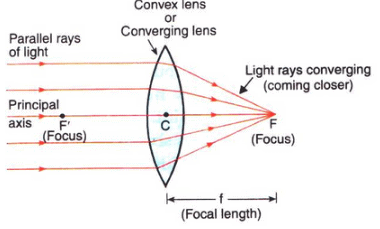(b) Principle axis: The principal axis of a lens is a line passing throught the optical centre of the lens and perpendicular to both the faces of the lens.
Principle focus: The principal focus of a convex lens is a point on its principal axis to which light rays parallel to the principal axis converge after passing through the lens.
Focal length: The distance of the principle focus from the optical center of a lens is called its focal length.

Question 18: (a) Explain with the help of a diagram, why the concave lens is also called a diverging lens.
(b) Define the principal focus of a concave lens.

Solution : (a) A concave lens is known as diverging lens because it diverges the parallel rays of light passing through it.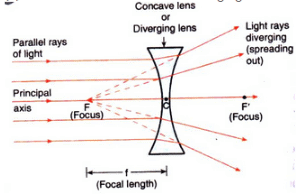(b) The principal focus of a concave lens is a point on its principal axis from which light rays, originally parallel to the axis, appear to diverge after passing through the lens.

Question 19: Draw a ray diagram to show the formation of a real magnified image by a convex lens. (In your sketch the position of object and image with respect to the principal focus of lens should be shown clearly).

Solution : Formation of real magnified image by a convex lens.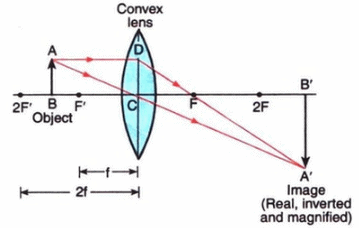Question 20: Describe with the help of a ray-diagram, the formation of image of a finite object placed in front of a convex lens between ƒ and 2ƒ Give two characteristics of the image so formed.

Solution : If object is placed in between f and 2f, the image will form on the other side of the lens beyond 2f as shown below.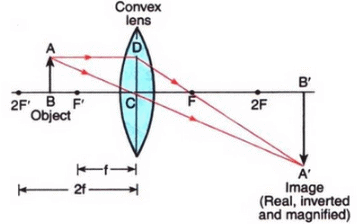Characteristics of image formed:
Image formed is real and inverted.
Image formed is magnified.

Question 21: Describe with the help of a ray diagram the nature, size and position of the image formed when an object is placed in front of a convex lens between focus and optical centre. State three characteristics of the image formed.

Solution : In the diagram, the object is placed in front of a convex lens between focus and optical centre. The image is formed on the same side as the object as shown below.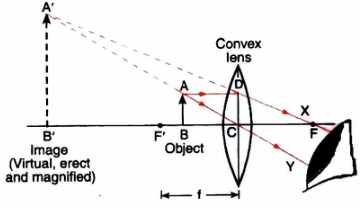Characteristics of image formed:
Image is virtual and erect.
Image is larger than the object
Image is formed behind the object.

Question 22: An object is placed at a distance equal to 2ƒ in front of a convex lens. Draw a labelled ray diagram to show the formation of image. State two characteristics of the image formed.

Solution :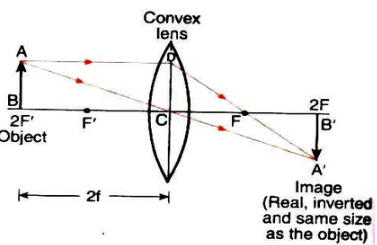Characteristic of image formed:
Image formed is real and inverted.
Image is of same size as the object

Question 23: Describe with the help of a ray-diagram, the size, nature and position of the image formed by a convex lens when an object is placed beyond 2f in front of the lens.

Solution : When an object is placed beyond 2f in front of a convex lens, then the image formed is between f and 2f on the other side of the lens, it is real, inverted and smaller than the object.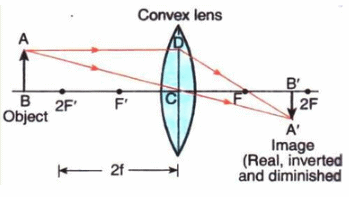Question 24: Describe with the help of a ray diagram the nature, size and position of the image formed when an object is placed at infinity (considerable distance) in front of a convex lens. State three characteristics of the image so formed.

Solution : When an object is placed at infinity in front of a convex lens, the image is formed at the focus on the other side of the lens.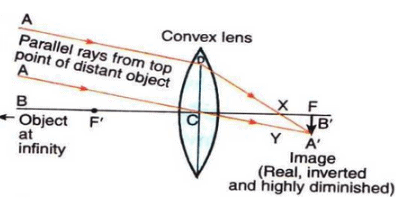Characteristics of image formed:
Image is real.
Image is inverted.
Image is highly diminished.

Question 25: (a) What type of lens is shown in the diagram on the right ? What will happen to the parallel rays of light ? Show by completing the ray diagram.
(b) Your eye contains a convex lens. Why is it unwise to look at the sun ?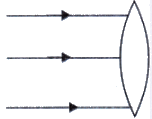Solution : (a) The lens shown in convex. the parallel rays will converge to a point called focus (F).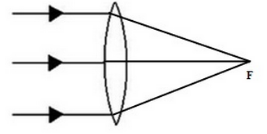(b) It is unwise to look at the sun because the convex lens focusses a lot of sun rays into our eyes and this may damage them.

Question 26: Where must the object be placed for the image formed by a converging lens to be :
(a) real, inverted and smaller than the object ?
(b) real, inverted and same size as the object ?
(c) real, inverted and larger than the object ?
(d) virtual, upright and larger than the object ?

Solution : a) Beyond 2F
b) At 2F
c) Between F and 2F
d) Between F and optical centre

Question 27: Draw a diagram to show how a converging lens held close to the eye acts as a magnifying glass. Why is it usual to choose a lens of short focal length for this purpose rather than one of long focal length ?

Solution : Converging lens as a magnifying glass: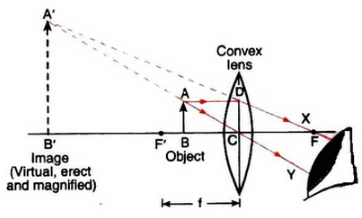It is usual to choose a lens of short focal length for this purpose rather than one of long focal length because smaller the focal length of a convex lens, greater will be its magnifying power.

Question 28: How could you find the focal length of a convex lens rapidly but approximately ?

Solution : To determine the focal length of a convex lens, we put the convex lens in a holder and keep it in front of a distant object like a window or tree, so that the rays coming from the window pass through it. A cardboard screen is put behind the lens. We change the distance of the screen from the convex lens until a clear inverted image of the window is formed on the screen. Measure the distance of the screen from the lens with a scale. This distance will be the focal length of convex lens.

Question 29: (a) With the help of a labelled diagram explain how a convex lens converges a beam of parallel light rays.
Mark the principal axis, optical centre, principal focus and focal length of the convex lens on the diagram.
(b) State whether convex lens has a real focus or a virtual focus.
(c) List some things that convex lens and concave mirror have in common.

Solution : (a) When a beam of light rays parallel to one another and also to the principal axis of the convex lens fall on the lens, the incident rays pass through the lens and get refracted according to the laws of refraction. All the rays, after passing through the lens, converge at the same point F (focus) on the other side of the lens.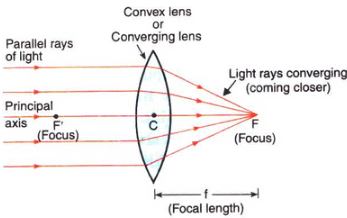(b) A convex lens has a real focus.
(c) Both, convex lens and concave mirror, converge parallel rays of light coming from infinity (parallel to the principal axis) at the focus.

Offer running on EduRev: Apply code STAYHOME200 to get INR 200 off on our premium plan EduRev Infinity!

94 docs

,

,

,

,

,

,

,

,

,

,

,

,

,

,

,

,

,

,

,

,

,

,

,

,

;